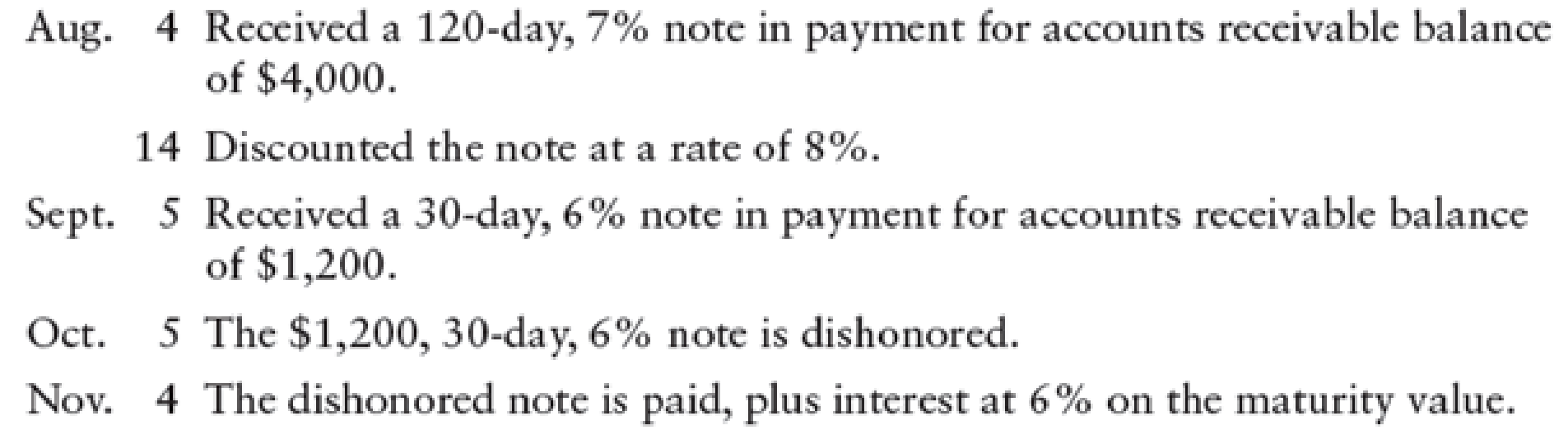Chapter 17, Problem 5SEB

Chapter
Section
Textbook Problem

JOURNAL ENTRIES (NOTE RECEIVED, DISCOUNTED, DISHONORED, AND COLLECTED) Prepare general journal entries for the following transactions:To determine

Prepare the journal entries to record the following transactions.

Explanation

Notes receivable:

Notes Receivable is a written promise to receive a certain amount on a future date, with certain percentage of interest. Companies use to issue notes receivable to meet short-term financing needs.

Prepare journal entry to record received 120-days, 7% note in payment for accounts receivable balance of $3,000.  Date Account titles and Explanation Debit Credit August 4 Notes receivable$4,000 Accounts receivable $4,000 (To record received note to settle account) Table (1) • Notes receivable is a current asset, and it is increased. Therefore, debit notes receivable account for$4,000.
• Accounts receivable is a current asset, and it is decreased. Therefore, credit accounts receivable account for $4,000. Prepare journal entry to record discounted the note at a rate of 8%.  Date Account titles and Explanation Debit Credit August 14 Cash$3,993.27 Interest expense (1) $6.73 Notes receivable (2)$4,000 (To record received payment of note with interest)

Table (2)

Working notes:

(1)Calculate cash proceeds.

Cash proceeds =Maturity value Discount amount (Difference in time period)=($4,000+($4,000×7%×120360))($4,093.33×8%×110360)=$4,093.33$100.06=$3,993.27

(2)Calculate interest revenue.

Interest expense =Maturity valueCash proceeds= $4,093.33$3,993.27=$6.73 • Cash is a current asset, and it is increased. Therefore, debit cash account for$3,993.27.
• Notes receivable is a current asset, and it is decreased. Therefore, credit notes receivable account for $4,000. • Interest expense is a component of stockholders’ equity, and it increases the expemse accounts. Therefore, debit interest expense account for$6.73.

Prepare journal entry to record received a 30-day, 6% note in payment for accounts receivable balance of $900.  Date Account titles and Explanation Debit Credit September 5 Notes receivable$1,200 Accounts receivable $1,200 (To record received note to settle account) Table (3) • Notes receivable is a current asset, and it is increased. Therefore, debit notes receivable account for$1,200.
• Accounts receivable is a current asset, and it is decreased. Therefore, credit accounts receivable account for $1,200. Prepare journal entry to record 30 day, 7% note is dishonored.  Date Account titles and Explanation Debit Credit October 5 Accounts receivable$1,206 Notes receivable $1,200 Interest revenue (3)$6 (To record notes receivable dishonored)

Table (4)

Working note:

(3)Calculate interest revenue

Still sussing out bartleby?

Check out a sample textbook solution.

See a sample solution

The Solution to Your Study Problems

Bartleby provides explanations to thousands of textbook problems written by our experts, many with advanced degrees!

Get Started

Find more solutions based on key concepts